# Simple Addition Permits Voltage Control Of DC-DC Converter's Output

In a standard dc-dc converter, a resistor divider typically defines a fixed output voltage. However, applications like programmable output voltage power supplies and motor control circuits require dynamic control of the dc-dc converter's output voltage. The circuit described here allows control of the converter's output voltage, VOUT, with a control voltage, VC.

In a conventional dc-dc buck converter, VOUT is: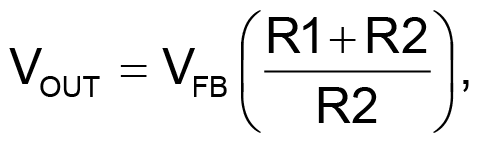(1)

so VOUT is fixed by the values of R1 and R2 (Fig. 1).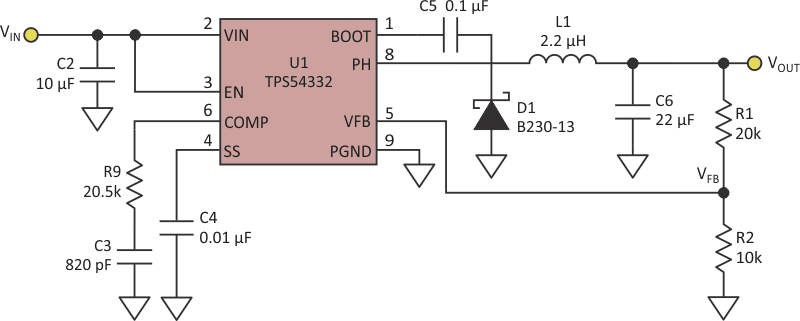Figure 1. The output voltage in a conventional dc-dc buck converter is fixed and depends on the resistor divider, R1/R2.

The added circuitry in Figure 2 enables users to control the same dc-dc converter's output voltage using VC.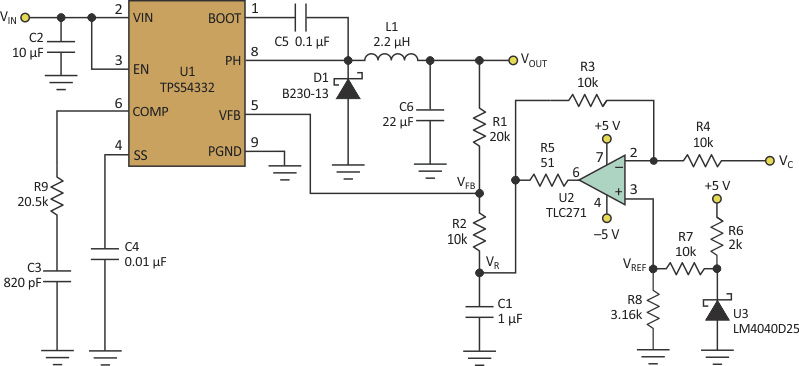Figure 2. The added circuitry in this version of the DC-DC converter permits control of VOUT by varying a control voltage, VC.

In this case, R2 is not connected to the ground but, rather, to VR. Equation 1 then becomes: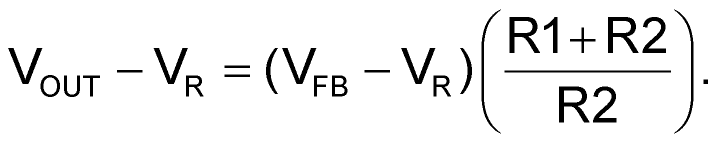(2)

Since R1 = 20 kΩ and R2 = 10 kΩ, Equation 2 can be simplified to: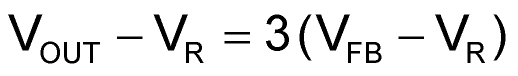(3)

or: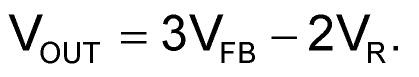(4)

R3 and R4 have the same value, 10 kΩ, so amplifier U2's output voltage is: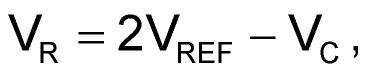(5)

where VREF is the reference voltage generated by U3 after resistor divider R7/R8.

Combining Equation 4 and Equation 5: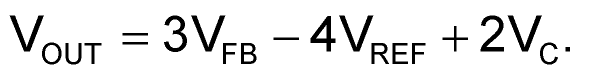(6)

To simplify Equation 6, choose components that make: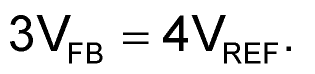(7)

Then Equation 6 becomes: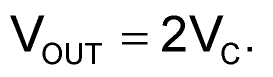(8)

electronicdesign.comYou may have to register before you can post comments and get full access to forum.
 User Name Remember Me? Password